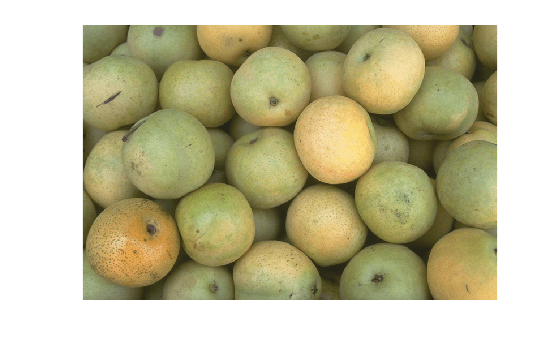Create binary mask image from ROI

## Syntax

``bw = createMask(ROI)``
``bw = createMask(ROI,m,n)``
``bw = createMask(ROI,I)``
``bw = createMask(ROI,hImage)``

## Description

example

``` `bw = createMask(ROI)` returns a binary mask image with pixels inside the ROI set to `true` and pixels outside the ROI set to `false`. ```
``` `bw = createMask(ROI,m,n)` returns a binary mask image that is size `[m,n]`.```
``` `bw = createMask(ROI,I)` returns a binary mask image that is the size of the image `I`.```
``` `bw = createMask(ROI,hImage)` returns a binary mask image that is the size of the Image object `hImage`.```

## Examples

collapse all

Read image into the workspace and display it.

```I = imread('pears.png'); imshow(I)```Draw an elliptical ROI on the image, using the `Center` parameter to specify the location of the ellipse and the`SemiAxes` parameter to specify the shape of the ellipse. The example also specifies that the edge of the ellipse is a striped line.

```h = drawellipse('Center',[447 204],'SemiAxes',[78 72], ... 'RotationAngle',287,'StripeColor','m');```Get a binary mask from the ROI. Pixels inside the ROI are `true` and pixels outside the ROI are `false`. Display the mask.

```mask = createMask(h); imshow(mask)```## Input Arguments

collapse all

Region of interest, specified as an ROI object of one of the following types:

Row dimension of the mask image, specified as a positive integer.

Column dimension of the mask image, specified as a positive integer.

Input image array, specified as a numeric array.

Input image object, specified as an Image object. For more details, see Image Properties.

## Output Arguments

collapse all

Binary mask image, returned as a logical array.

Data Types: `logical`

## Tips

• For more information about classifying pixels on the ROI boundary, see Classify Pixels That Are Partially Enclosed by ROI.

• If you specify a mask size that does not match the size of the image associated with the `ROI` object, then `createMask` crops or zero-pads the mask to the specified size. The image associated with the ROI object is `ROI.Parent.Children(2,1).CData`.

## Version History

Introduced in R2018b